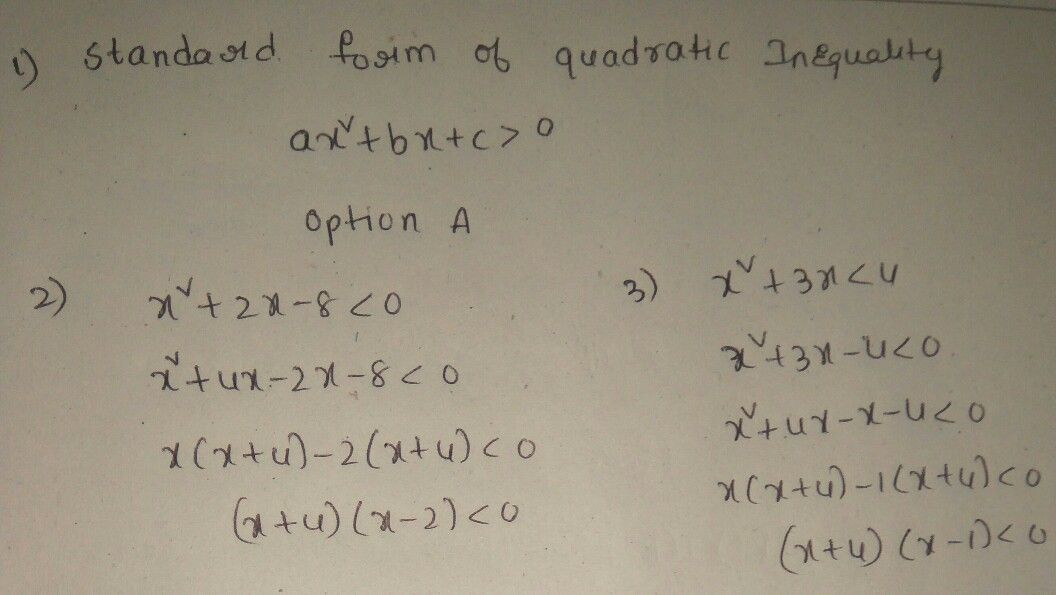Symbol
Problemanswer sheet. 1. What is the standard $form$ of quadratic inequality? A. $x^{2}+bx+c>0$ B. $ax^{2}+bx+c=$ = 0 C. $Ax+By$ $+C=0$ D. $Bx+C<0$ 2 In quadratic inequality $x^{2+2x-8}<0$ which of the following solutions or roots makes inequality true? A. $4<x>1$ B. $-4<x<2$ C. $-4<x$ $4<x<3$ D. $-4>x>3$ . What is the solution set of the quadratic inequality $x^{2}+3x<47$ $-4<x<1$ B. $-4<x<2$ $A.$ $c.$ $-4<x<3$ D. $-4>x>3$
7th-9th grade
Geometry
Search count: 107
SolutionQanda teacher - saikumarpls provide extra gift coins